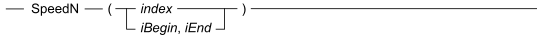# 16.20 - Syntax - Teradata Vantage NewSQL Engine

## Teradata Vantage™ Geospatial Data Types

prodname
vrm_release
16.20
created_date
March 2019
category
Programming Reference
featnum
B035-1181-162Kindex
An INTEGER for the index of the point within the GeoSequence type, where the index of the first point is 1.
iBegin
An INTEGER for the index of the starting point for which to calculate the speed, where the index of the first point in a GeoSequence type is 1.
iEnd
An INTEGER for the index of the ending point for which to calculate the speed, where the index of the first point in a GeoSequence is 1.